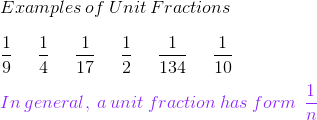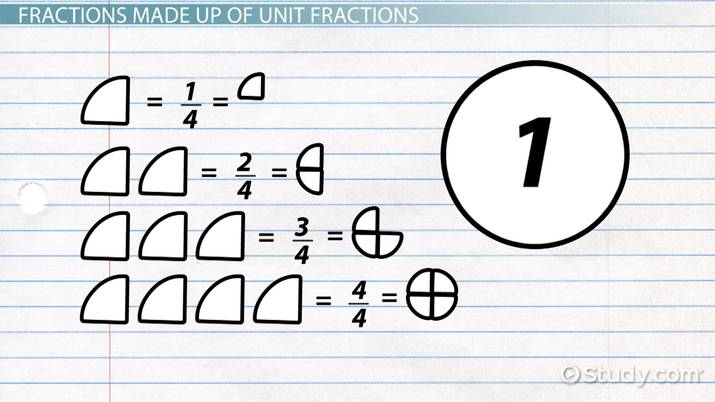# What is a Unit Fraction? - Definition & Examples

Lesson Transcript
Instructor: Laura Pennington

Laura received her Master's degree in Pure Mathematics from Michigan State University, and her Bachelor's degree in Mathematics from Grand Valley State University. She has 20 years of experience teaching collegiate mathematics at various institutions.

In this lesson, we'll define unit fractions. We'll use definitions and examples to illustrate how these types of fractions are parts of the number 1 and how they can be used to make up other fractions.

## Unit Fractions

What is your favorite flavor of pie? Is it apple, blueberry, or pumpkin? Suppose you just baked up your favorite pie. You're probably anxious to dig in and eat it, but before you do, let's use it to explain unit fractions!

A unit fraction is a fraction with a numerator equal to 1. For example, this image shows unit fractions:In general, 1/n is a unit fraction.An error occurred trying to load this video.

Try refreshing the page, or contact customer support.

Coming up next: Comparing and Ordering Fractions

### You're on a roll. Keep up the good work!

Replay
Your next lesson will play in 10 seconds
• 0:03 Unit Fractions
• 0:31 Unit Fractions: Part…
• 1:53 Fractions Made Up of…
• 3:15 Example
• 4:09 Lesson Summary
Save Save

Want to watch this again later?

Timeline
Autoplay
Autoplay
Speed Speed

## Unit Fractions: Part of the Number One

When it comes to unit fractions, each unit fraction is a part of the number 1. For example, 1/2 is a half of 1, 1/3 is a third of 1, 1/4 is a fourth of 1, and so on. To illustrate this, let's consider your pie.

Let your pie represent the number 1. If you slice the pie into n equal sized slices, then you split the pie, or 1, into n equal parts, where each slice is a part of the pie, or a part of 1. For instance, if we cut the pie into 6 equal slices, then 1 slice represents 1/6 of the whole pie. In the same way, if we break 1 into 6 equal parts, 1 part represents 1/6 of 1.

Unit fractions are 1 part of the number 1. Notice that the more pieces you slice the pie into, the smaller the pieces of pie become. This tells us that the larger the denominator of a unit fraction, the smaller the part of 1 it represents.

Let's break this down even further to solidify our understanding of these types of fractions. In general, a fraction's denominator tells us the number of parts we are breaking the whole into. Since the numerator's always 1 in unit fractions, the denominator tells us how many parts you're breaking 1 into, and you call these parts units. Therefore, the denominator of a unit fraction tells us the unit.

## Fractions Made Up of Unit Fractions

You're probably getting a little impatient now because you want to eat your pie, but let's illustrate a few more things. Notice that if you break the pie into n equal slices and then take a certain number of those slices, let's say m slices, those m slices represent m/n. In the same way, if we break 1 into n equal parts and then take m parts, we have m/n of 1. This fact illustrates that all fractions are made up of unit fractions.

To unlock this lesson you must be a Study.com Member.

### Register to view this lesson

Are you a student or a teacher?